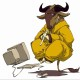# dud3

Member

12

102 Neutral

• Rank
Member

• Interests
Programming

## Recent Profile Visitors

The recent visitors block is disabled and is not being shown to other users.

1. This is the current code, under dragTPSInput(...): #pragma once #include <glm.hpp> #include <gtc/matrix_transform.hpp> #include <gtc/type_ptr.hpp> #include "InputHandler.h" #include "Vector2D.h" class Camera { public: glm::vec3 m_position; glm::vec3 m_front; glm::vec3 m_up; glm::vec3 m_right; glm::vec3 m_worldup; float m_yaw; float m_pitch; float m_zoom; bool m_enableInput; // Mouse float m_mouseSensitivity; enum class direction { in, out, right, left }; Camera(glm::vec3 position = glm::vec3(0.0f, 0.0f, 0.0f), glm::vec3 up = glm::vec3(0.0f, 1.0f, 0.0f), float yaw = -90.0f, float pitch = 0.0, float zoom = 1.0f, float mouseSensitivity = 0.05f) : m_front(glm::vec3(0.0f, 0.0f, -1.0f)), m_enableInput(false) { m_position = position; m_yaw = yaw; m_pitch = pitch; m_worldup = up; m_zoom = zoom; m_mouseSensitivity = mouseSensitivity; updateCameraVectors(); } void lookAt() { } void move(direction d) { if (d == direction::in) m_position -= m_front; if (d == direction::out) m_position += m_front; if(d == direction::right) m_position += glm::normalize(glm::cross(m_front, m_up)) * m_mouseSensitivity; if(d == direction::left) m_position -= glm::normalize(glm::cross(m_front, m_up)) * m_mouseSensitivity; } void rotate() { } void zoom() { } void scrollInput() { } void keyboardInput() { // todo: give the user ability to adjust input keys if (_inHandler->onKeyDown(SDL_SCANCODE_W)) { move(direction::in); } if (_inHandler->onKeyDown(SDL_SCANCODE_S)) { move(direction::out); } if (_inHandler->onKeyDown(SDL_SCANCODE_D)) { move(direction::right); } if (_inHandler->onKeyDown(SDL_SCANCODE_A)) { move(direction::left); } } void dragFPSInput(Vector2D* mouseMoveDiff, bool constrainPitch = true) { m_yaw += mouseMoveDiff->getX(); // offsetx m_pitch += -(mouseMoveDiff->getY()); // offsety // Make sure that when pitch is out of bounds, screen doesn't get flipped if (constrainPitch) { if (m_pitch > 89.0f) m_pitch = 89.0f; if (m_pitch < -89.0f) m_pitch = -89.0f; } // Update Front, Right and Up Vectors using the updated Eular angles updateCameraVectors(); } void dragTPSInput(Vector2D* mouseMoveDiff) { // m_position = glm::rotate(m_position, -mouseMoveDiff->getY() * m_mouseSensitivity, glm::vec3(1, 0, 0)); // m_position = glm::rotate(m_position, -mouseMoveDiff->getX() * m_mouseSensitivity, glm::vec3(0, 1, 0)); glm::quat rot = glm::angleAxis(glm::radians(-mouseMoveDiff->getY()), glm::vec3(1, 0, 0)); rot = rot * glm::angleAxis(glm::radians(-mouseMoveDiff->getX()), glm::vec3(0, 1, 0)); glm::mat4 rotMatrix = glm::mat4_cast(rot); glm::vec4 pos = glm::vec4(m_position.x, m_position.y, m_position.z, 1.0f); pos = rotMatrix * pos; m_position.x = pos.x; m_position.y = pos.y; m_position.z = pos.z; updateCameraVectors(); } void onInput(bool drag = true, bool scroll = true, bool keyboard = false) { if (drag) if (_inHandler->getMouseButtonState(_inHandler->mouse_buttons::LEFT)) if(_inHandler->isMouseMovig()) dragTPSInput(_inHandler->getMouseMoveDiff()); if (scroll) scrollInput(); if (keyboard) keyboardInput(); } // Returns the view matrix calculated using Eular Angles and the LookAt Matrix glm::mat4 getViewMatrix() { glm::vec3 pos = glm::vec3(m_position.x, m_position.y, m_position.z); return glm::lookAt(pos, m_front, m_up); } private: void updateCameraVectors() { // Calculate the new Front vector glm::vec3 front; front.x = cos(glm::radians(m_yaw)) * cos(glm::radians(m_pitch)); front.y = sin(glm::radians(m_pitch)); front.z = sin(glm::radians(m_yaw)) * cos(glm::radians(m_pitch)); m_front = glm::normalize(front); // Also re-calculate the Right and Up vector // Normalize the vectors, because their length gets closer to 0 the more you look up or down which results in slower movement. m_right = glm::normalize(glm::cross(m_front, m_worldup)); m_up = glm::normalize(glm::cross(m_right, m_front)); } InputHandler* _inHandler = TheInputHandler::Instance(); };
2. I think I'm stuck, I'm just trying to achieve the same rotation around the x axis as in blender. The video shows the difference between blender and my rotation:
3. How do we rotate the camera around x axis 360 degrees, without having the strange effect as in my video below? Mine behaves exactly the same way spherical coordinates would, I'm using euler angles. Tried googling, but couldn't find a proper answer, guessing I don't know what exactly to google for, googled 'rotate 360 around x axis', got no proper answers. References: Code: https://pastebin.com/Hcshj3FQ The video shows the difference between blender and my rotation:
4. Indeed you right, that statement is irrelevant, as I've mentioned I'm not very familiar with C++, and since SDL is written in C, I tend to write C like C++.
5. I wouldn't use C++ stuff on SDL, such as the one mentioned on my last comment, I also think you most likely didn't include the SDL header file, or didn't set the correct compiler flags, or both.
6. I'm not very familiar with C++ but why are you passing reference to pointer SDL_Renderer*& instead of SDL_Renderer* pointer to struct?
7. I think you won't be using more than 5-6 git shell commands, if you decide using git. Also github provides you useful GUI tools: issue tracker, pull request manager... on top of that github provides you a GUI version of git commands, if you don't want to touch the shell. Finally you have tons of github integrations(travis ci, appveyor...).
8. Sounds like a nice idea, I'd just have to find the vertices and then the convex hull, I wanted something alike the bounding box on my images, but I think your idea could solve my problem as well.
9. Yes that's a great idea, indeed implementing the adaptive algorithm would take more time than 4-5 hours. I'll fix the issue the way you suggested, and then try writing the adaptive algorithm just as a training for myself. Thanks.
10. I see, I guess I would have to develop an adaptive algorithm and train it with lots and lots of data...
11. Unfortunately I don't have additional data on this image, actually there are 100s of similar images, I could manually set their bounds, but that would take quite some time, also I think it would be fun to find (if any) way to find the bounding. I'm thinking we could find the exact bonds of this image from it's pixel data and then guide it using isometric axis (in case there are complicated shape), just trying to come up with a conceptual idea currently, unsure how possible it is to solve this problem.
12. Has anyone tried finding isometric bounds programatically? What I'm trying to achieve is to use the Cartesian width and height of the object to find it's isometric bounds, but I think that just doesn't work once the image gets complicated. The attachments should explain clearly what I'm trying to achieve (note: the bounds drawing isn't perfect).